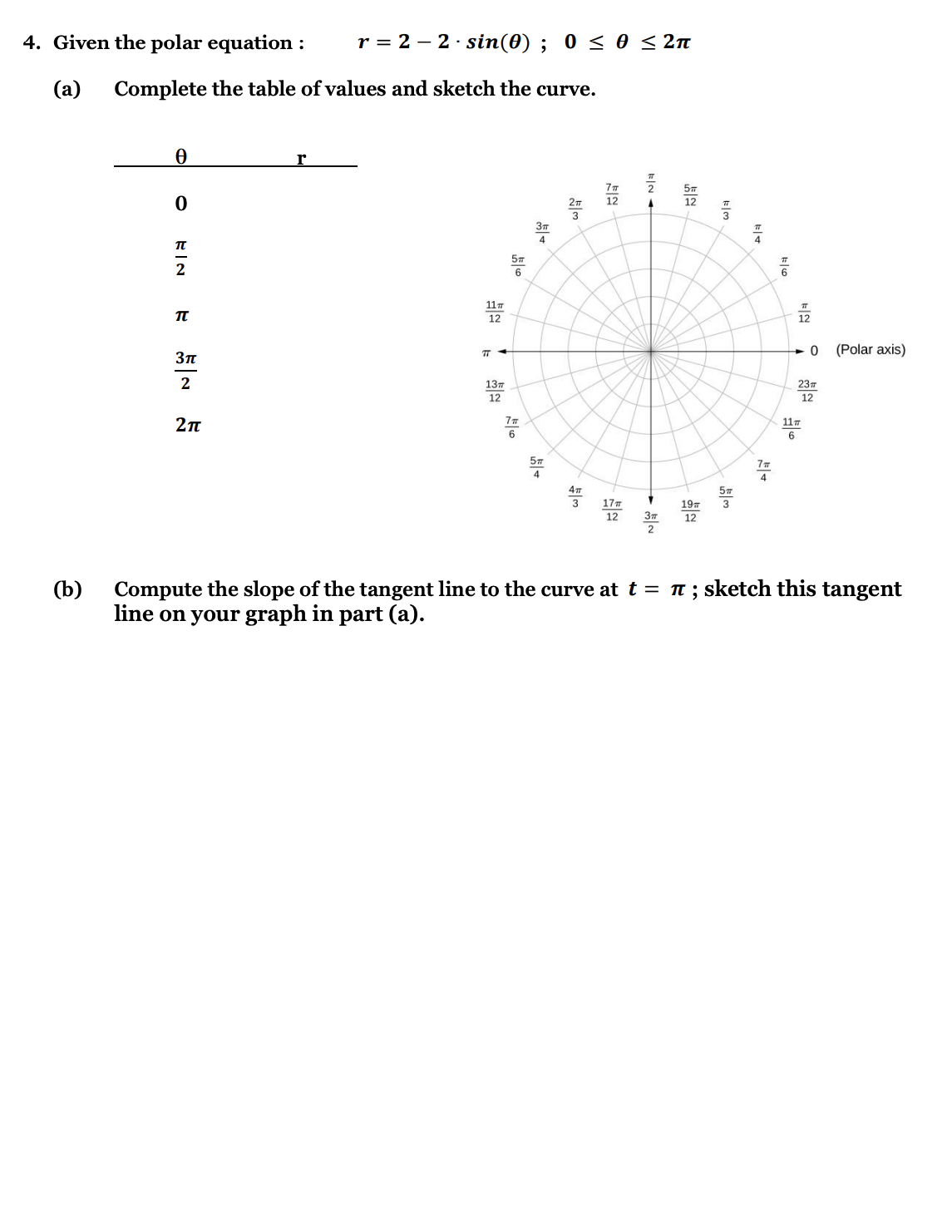YY

Yaboiimarco Y.

Calculus 2 / BC

5 days, 11 hours ago

All problems step by stepAll problems step by step

### Discussion

You must be signed in to discuss.

### Video Transcript

this problem assets to solve for the slope of the tangent line at a given point for this polar equation. So the first step is we can take the derivative of the equation D R over defeat up, which will equal applying the chain rule. We take out the two. So yet for co sign to theater as a derivative. Next, we can use the equation for the slope of the Tangela and D y over DX is equal to d r o ver di fada sine theta plus our coastline data divided by D. R D Theta Cho Sang Veda minus our science data and plugging in DRV theater. This will become for co sign to theta Sign data plus our co Cynthia, divided by four coastline tooth data coastline beta minus our scientist. Next, the point that the problem asked us to solve. That is, at three Piper for so we can plug this into our equation for do, however DX, and that gives us do already. X is equal to four Times co sign times two times prior for which is equal to pi over two time sign pile core plus three co sign Pi over four divide by four co sign pyre for two again co sign pyre before minus three. Sign plant before when we see that these equals zero here and that co some power for and signed pyre for are the same article the same value. So savings out, we get that the slope of the tangent line is equal to negative one.##### Top Calculus 2 / BC Educators##### Heather Z.

Oregon State University##### Kristen K.

University of Michigan - Ann Arbor